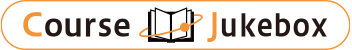### CourseDetailDegree
Bachelor
1
Course delivery methods
face-to-face
Subject
Physical sciences, Mathematical sciences, Engineering & technology
Program
School
School of Science and Engineering
Department
Bachelor's Program in Interdisciplinary Engineering
Campus
Tsukuba Campus
Classroom
3A405
Course Offering Year
2023-2024
Course Offering Month
October - December
January - February
Weekday and Period
Thu2 Thu1,2
Capacity
Credits
2.0
Language
English
Course Number
FJ20201

### Probability and Statistics University of Tsukuba

#### Course Overview

This course introduces basics of probability theory and statistics. This course will be mainly oriented to interpret physical problems in engineering and natural sciences through application of probability theory and statistics. Evaluation will be done through class quiz, homework on regular basis, and final examinations.

#### Learning Achievement

This course introduces basics of probability theory and statistics. This course will be mainly oriented to interpret physical problems in engineering and natural sciences through application of probability theory and statistics. Evaluation will be done through class quiz, homework on regular basis, and final examinations.

#### Competence

Related to 1. Mathematical logic and calculation skills.

#### Course prerequisites

In addition to high-school algebra, a preliminary skill in calculus is needed.

Class Quiz+ Homework 60%
Final Exam 40%

#### Course schedule

Introduction, Set Theorem
Outcomes, events, and probability
Conditional probability and independence
Discrete random, random variables
Continuous random variables
Simulation
Joint distributions and independence
The Poisson process
Gaussian distribution
Data distributions, mean, median, mode, variance

Lectures